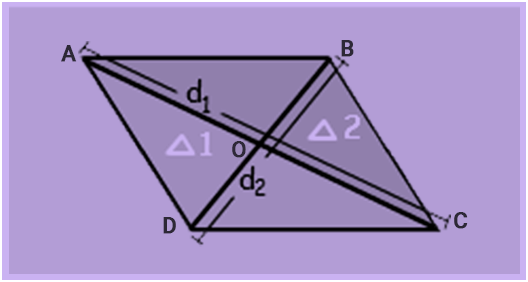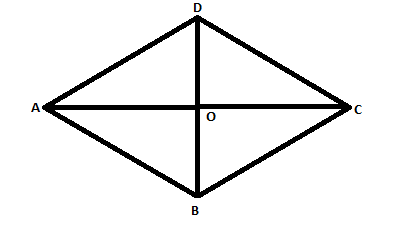# Area of a Rhombus Formula

With the resemblance of diamond, a rhombus is a flat figure with four equal straight sides. All sides of the rhombus have equal length, opposite sides are parallel, and opposite angles are equal. Another name for rhombus is equilateral quadrilateral, means that all of its sides are equal in length. A rhombus is in the shape of a slanting square. The area of the rhombus is half the product of the diagonals.The Area of a Rhombus Formula is,
4 × area of ∆ AOB
= 4 × $\frac{1}{2}$ × AO × OB sq. units
= 4 × $\frac{1}{2}$ × $\frac{1}{2}$ $d_{1}$ × $\frac{1}{2}$ $d_{2}$ sq. units
= 4 × $\frac{1}{8}$ $d_{1}$ × $d_{2}$ square units
= $\frac{1}{2}$ × $d_{1}$ × $d_{2}$

Therefore
Where, Area of a Rhombus = A = $\frac{1}{2}$ × $d_{1}$ × $d_{2}$
$d_{1}$ and $d_{2}$ are the diagonals of the rhombus.

### Solved Examples

Question 1: Find the area of the rhombus having each side equal to 17 cm and one of its diagonals equal to 16 cm.
Solution:ABCD is a rhombus in which AB = BC = CD = DA = 17 cm
Diagonal AC = 16 cm (with O being the diagonal intersection point)
Therefore, AO = 8 cm
In ∆ AOD,
$AD^{2}$ = $AO^{2}$ + $OD^{2}$
$17^{2}$ = $8^{2}$ + $OD^{2}$
289 = 64 + $OD^{2}$
225 = $OD^{2}$
OD = 15
Therefore, BD = 2 $\times$ OD
= 2 $\times$ 15
= 30 cm
Now, area of rhombus
= $\frac{1}{2}$ $\times$ $d_{1}$ $\times$ $d_{2}$
= $\frac{1}{2}$ $\times$ 16 $\times$ 30
= 240 $cm^{2}$